## Quick Sort Back

### Overview

• 快速排序: 分治思想(Divide, Conquer and Combination)
• Divide: pivot(可隨機選定)
• Conquer: 直到只有一個的時候合併
• Combination: None
• 时间复杂度:
•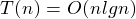(最好平均情況)
•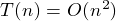(最壞情況)
• 空間複雜度: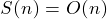• 稳定性: 不稳定
• 适用情况: 数据多的且無序
• 最壞情況下的比較次數: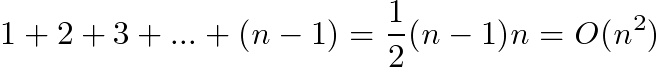### Example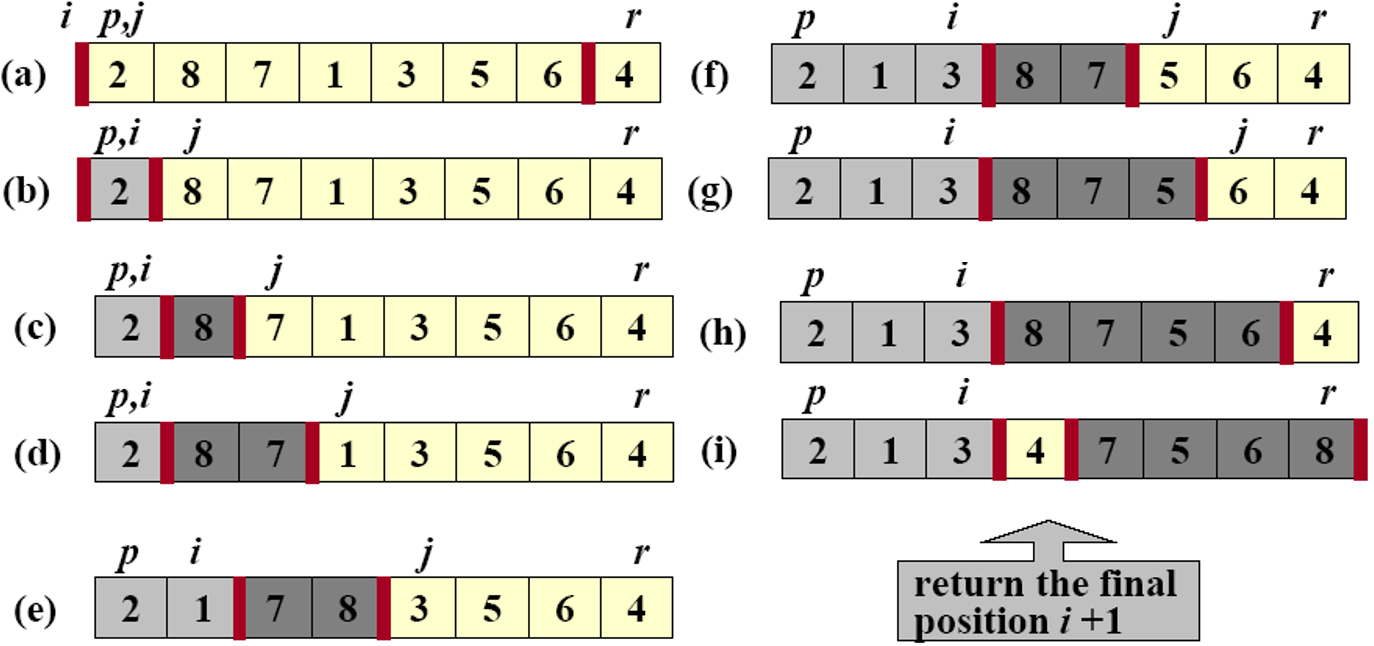### Code

``````void QUICKSORT(int A[], int p, int q)
{
if (p < q)
{
int m = PARTITION(A, p, q);
QUICKSORT(A, p, m - 1);
QUICKSORT(A, m + 1, q);
}
}

int PARTITION(int A[], int p, int q)
{
int x = A[q];        //pivot
int i = p - 1;
for (int j = p; j <= q - 1; j++)
{
count++;
if (A[j] <= x)
{
i++;
exchange(A, i, j);
}
}
exchange(A, i + 1, q);
return i + 1;
}
``````